# Hashing (Hash table, Hash functions and its characteristics)

In this article, we are going to study about Hashing, Hash table, Hash function and the types of hash function. Characteristics of good hash function and collision resolution technique are also prescribed in this article.
Submitted by Abhishek Kataria, on June 21, 2018

## Hashing

There are many possibilities for representing the dictionary and one of the best methods for representing is hashing. Hashing is a type of a solution which can be used in almost all situations. Hashing is a technique which uses less key comparisons and searches the element in O(n) time in the worst case and in an average case it will be done in O(1) time. This method generally used the hash functions to map the keys into a table, which is called a hash table.

1) Hash table

Hash table is a type of data structure which is used for storing and accessing data very quickly. Insertion of data in a table is based on a key value. Hence every entry in the hash table is defined with some key. By using this key data can be searched in the hash table by few key comparisons and then searching time is dependent upon the size of the hash table.

2) Hash function

Hash function is a function which is applied on a key by which it produces an integer, which can be used as an address of hash table. Hence one can use the same hash function for accessing the data from the hash table. In this the integer returned by the hash function is called hash key.

## Types of hash function

There are various types of hash function which are used to place the data in a hash table,

1. Division method

In this the hash function is dependent upon the remainder of a division. For example:-if the record 52,68,99,84 is to be placed in a hash table and let us take the table size is 10.

Then:

```h(key)=record% table size.
2=52%10
8=68%10
9=99%10
4=84%10
```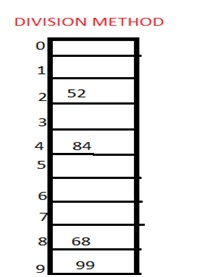2. Mid square method

In this method firstly key is squared and then mid part of the result is taken as the index. For example: consider that if we want to place a record of 3101 and the size of table is 1000. So 3101*3101=9616201 i.e. h (3101) = 162 (middle 3 digit)

3. Digit folding method

In this method the key is divided into separate parts and by using some simple operations these parts are combined to produce a hash key. For example: consider a record of 12465512 then it will be divided into parts i.e. 124, 655, 12. After dividing the parts combine these parts by adding it.

```    H(key)=124+655+12
=791
```

### Characteristics of good hashing function

1. The hash function should generate different hash values for the similar string.
2. The hash function is easy to understand and simple to compute.
3. The hash function should produce the keys which will get distributed, uniformly over an array.
4. A number of collisions should be less while placing the data in the hash table.
5. The hash function is a perfect hash function when it uses all the input data.

### Collision

It is a situation in which the hash function returns the same hash key for more than one record, it is called as collision. Sometimes when we are going to resolve the collision it may lead to a overflow condition and this overflow and collision condition makes the poor hash function.

### Collision resolution technique

If there is a problem of collision occurs then it can be handled by apply some technique. These techniques are called as collision resolution techniques. There are generally four techniques which are described below.

1) Chaining

It is a method in which additional field with data i.e. chain is introduced. A chain is maintained at the home bucket. In this when a collision occurs then a linked list is maintained for colliding data.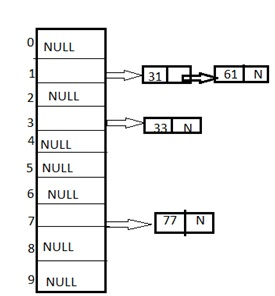Example: Let us consider a hash table of size 10 and we apply a hash function of H(key)=key % size of table. Let us take the keys to be inserted are 31,33,77,61. In the above diagram we can see at same bucket 1 there are two records which are maintained by linked list or we can say by chaining method.

2) Linear probing

It is very easy and simple method to resolve or to handle the collision. In this collision can be solved by placing the second record linearly down, whenever the empty place is found. In this method there is a problem of clustering which means at some place block of a data is formed in a hash table.

Example: Let us consider a hash table of size 10 and hash function is defined as H(key)=key % table size. Consider that following keys are to be inserted that are 56,64,36,71.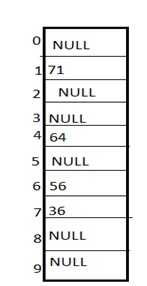In this diagram we can see that 56 and 36 need to be placed at same bucket but by linear probing technique the records linearly placed downward if place is empty i.e. it can be seen 36 is placed at index 7.

This is a method in which solving of clustering problem is done. In this method the hash function is defined by the H(key)=(H(key)+x*x)%table size. Let us consider we have to insert following elements that are:-67, 90,55,17,49.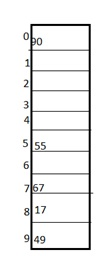In this we can see if we insert 67, 90, and 55 it can be inserted easily but at case of 17 hash function is used in such a manner that :-(17+0*0)%10=17 (when x=0 it provide the index value 7 only) by making the increment in value of x. let x =1 so (17+1*1)%10=8.in this case bucket 8 is empty hence we will place 17 at index 8.

4) Double hashing

It is a technique in which two hash function are used when there is an occurrence of collision. In this method 1 hash function is simple as same as division method. But for the second hash function there are two important rules which are

1. It must never evaluate to zero.
2. Must sure about the buckets, that they are probed.

The hash functions for this technique are:

```    H1(key)=key % table size
H2(key)=P-(key mod P)
```

Where, p is a prime number which should be taken smaller than the size of a hash table.

Example: Let us consider we have to insert 67, 90,55,17,49.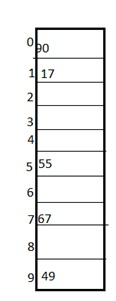In this we can see 67, 90 and 55 can be inserted in a hash table by using first hash function but in case of 17 again the bucket is full and in this case we have to use the second hash function which is H2(key)=P-(key mode P) here p is a prime number which should be taken smaller than the hash table so value of p will be the 7.

i.e. H2(17)=7-(17%7)=7-3=4 that means we have to take 4 jumps for placing the 17. Therefore 17 will be placed at index 1.

Top MCQs